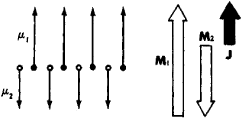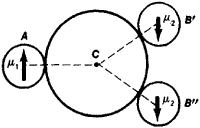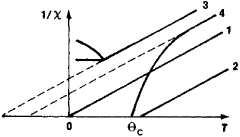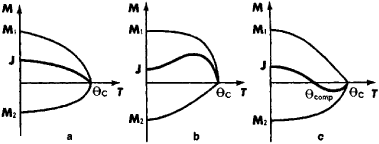# Ferrimagnetism

(redirected from Ferrimagnetic)
Also found in: Dictionary, Thesaurus, Wikipedia.

## Ferrimagnetism

A specific type of ordering in a system of magnetic moments or the magnetic behavior resulting from such order. In some magnetic materials the magnetic ions in a crystal unit cell may differ in their magnetic properties. This is clearly so when some of the ions are of different species. It is also true for similar ions occupying crystallographically inequivalent sites. Such ions differ in their interactions with other ions, because the dominant exchange interaction is mediated by the neighboring nonmagnetic ions. They also experience different crystal electric fields, and these affect the magnetic anisotropy of the ion. A collection of all the magnetic sites in a crystal with identical behavior is referred to as a magnetic sublattice. A material is said to exhibit ferrimagnetic order when, first, all moments on a given sublattice point in a single direction and, second, the resultant moments of the sublattices lie parallel or antiparallel to one another. The notion of such an order is due to L. Néel, who showed in 1948 that its existence would explain many of the properties of the magnetic ferrites. See Ferromagnetism

In general, there is a net moment, the algebraic sum of the sublattice moments, just as for a normal ferromagnet. However, its variation with temperature rarely exhibits the very simple behavior of the normal ferromagnet. For example, in some materials, as the temperature is raised over a certain range, the magnetization may first decrease to zero and then increase again. Ferrimagnets can be expected, in their bulk properties, measured statically or at low frequencies, to resemble ferromagnets with unusual temperature characteristics. See Curie temperature

## Ferrimagnetism

a magnetic state of a substance, wherein the magnetic moments of the ions composing the substance (a ferrimagnet) form two or more subsystems called magnetic sub-lattices. Each sublattice contains ions of one kind with identically oriented magnetic moments. The magnetic moments of ions of different sublattices are nonparallel and, in the more general case, form a complex three-dimensional configuration, for example, a triangular configuration. The number of ions in one sublattice is often a multiple of the number of ions in another. The simplest model of ferrimagnetic ordering is shown in Figure 1. The spontaneous magnetization J of a ferrimagnetic substance is equal to the vector sum of the magnetizations of all the sublattices.Figure 1. Schematic diagram of the ferrimagnetic ordering of a linear chain of magnetic ions of different kinds with magnetic moments μ1 and μ2. M1 = Nμ1 and M2 = Nμ2 are the magnetizations of the first and second sublattices. The total magnetization is J = M1M2.

Ferrimagnetism may be considered the most general case of a magnetically ordered state. From this viewpoint, ferromagnetism is a particular case of ferrimagnetism in which a substance contains only one sublattice, and antiferromagnetism is a particular case in which all sublattices consist of identical magnetic ions and J = 0. The term “ferrimagnetism” was introduced by L. Néel in 1948 and is derived from the word “ferrite,” which is the name of a large class of oxides of the transition elements in which the phenomenon was first observed.

A necessary condition for ferrimagnetism is the presence in a substance of positive ions, or cations, with an intrinsic magnetic moment that are formed from elements having an unfilled d or f electron shell. A negative exchange interaction that tends to align the magnetic moments antiparallel must occur between the ions of different sublattices. As a rule, the interaction is a superexchange interaction; that is, it occurs by means of the exchange of electrons through an intermediate nonmagnetic anion, for example, an oxygen ion (Figure 2).Figure 2. A typical arrangement of the ions in a ferrimagnetic crystal: (C) a nonmagnetic anion; (A), (B′), and (B″) magnetic cations of the first and second sublattices. The main superexchange interaction between A and B′, B″ is negative. The interaction B′–B″ is insignificant.

At high temperatures, where the energy of thermal motion is much greater than the exchange energy, a substance has paramagnetic properties (seePARAMAGNETISM). The temperature dependence of the magnetic susceptibility of paramagnets, in which ferrimagnetism occurs at low temperatures, has the characteristic features shown in Figure 3. The reciprocal susceptibility (1/χ) of paramagnetic substances obeys the Curie-Weiss law with a negative constant θ = Δ at high temperatures and sharply decreases with decreasing temperature, tending to zero as T → θC. At the Curie point θC, where the energy of the exchange interaction becomes equal to the energy of thermal motion in the substance, ferrimagnetic ordering occurs. In most cases, the transition to the ordered state is a second-order phase transition and is accompanied by characteristic anomalies of specific heat, linear expansion, and galvanomagnetic and other properties.

The ferrimagnetic ordering of moments that occurs is described by a certain magnetic structure, that is, by the division of a crystal into magnetic sublattices and by the magnitude and direction of the sublattice magnetization vectors. The magnetic structure can be determined by neutron-diffraction techniques (seeDIFFRACTION OF PARTICLES). The formation of a given magnetic structure depends on the crystal structure of the substance and the ratio of the magnitudes of the exchange interactions between different magnetic ions. The exchange interaction determines only the orientation of the sublattice magnetizations relative to each other. Another parameter—the orientation relative to the crystallographic axes—is determined by the magnetic-anisotropy energy, which is several orders of magnitude less than the exchange energy.Figure 3. The temperature dependence of the reciprocal magnetic susceptibility 1/χ: (1) a paramagnet with χ = C/T, (2) a ferromagnet with χ = C/(T–θ), (3) an antiferromagnet with χ = C/(T + θ), (4) a ferrimagnet

The existence of several different sublattices in a ferrimagnet leads to a more complex temperature dependence of the spontaneous magnetization J than in an ordinary ferromagnet. Such a temperature dependence occurs because the temperature dependences of the magnetization of each sublattice may differ (Figure 4). As a result, as the temperature increases from absolute zero, the spontaneous magnetization, which is the difference between the sublattice magnetizations in the simplest case, may: (1) decrease monotonically (Figure 4,a), as in an ordinary ferromagnet; (2) increase at low temperatures and thereafter pass through some maximum (Figure 4,b); or (3) tend to zero at some specified temperature θcomp. The temperature θcom is called the compensation point. At T > θcomp or T < θcomp, the spontaneous magnetization is nonzero.

A theoretical description of the properties of ferrimagnets was first given in 1948 by Néel, who demonstrated that the major characteristics of the behavior of ferrimagnets may be explained very well in the framework of molecular-field theory. Ferrimagnets in magnetic fields that are much weaker than the exchange fields behave like ferromagnets, since such magnetic fields do not alter the magnetic structure. In the absence of a magnetic field, ferrimagnets are divided into domains and have a characteristic magnetization curve with saturation and hysteresis. Magnetostriction is observed in ferrimagnets. In ferrimagnets with noncol-linear magnetic structures, saturation is usually not observed at attainable magnetic-field values. Ferrimagnets have peculiar magnetic properties near the compensation point, where even weak magnetic fields cause the sublattices to slope and flip relative to each other. Far from the compensation point, such changes in magnetic structure occur in strong magnetic fields, that is, magnetic fields of the same order as the exchange fields. Under certain conditions, resonance absorption of electromagnetic energy is observed in ferrimagnets; such absorption is called ferrimagnetic resonance.Figure 4. Different types of temperature dependence of the sublattice magnetizations M1 and M2 and the spontaneous magnetization J for a ferrimagnet with two magnetic sublattices

The study of ferrimagnetism evolved very rapidly and further advanced the physics of magnetic phenomena. A theory of ferrimagnetic dielectrics (most ferrimagnets are dielectrics) has been developed. Many magnetic dielectrics are used widely in radio engineering, microwave engineering, and computer technology.

### REFERENCES

Smit, J., and Wijn, H. Ferrity. Moscow, 1962. (Translated from English.)
Redkozemel’nye ferromagnetiki i antiferromagnetiki. Moscow, 1965.
Gurevich, A. G. Magnitnyi rezonans v ferritakh i antiferromagnetikakh. Moscow, 1973.
Smolenskii, G. A., and V. V. Lemanov. Ferrity i ikh tekhnicheskoe primenenie. Leningrad, 1975.

A. S. BOROVIK-ROMANOV

## ferrimagnetism

[‚fe·ri′mag·nə‚tiz·əm]
(solid-state physics)
A type of magnetism in which the magnetic moments of neighboring ions tend to align nonparallel, usually antiparallel, to each other, but the moments are of different magnitudes, so there is an appreciable resultant magnetization. Also known as Néel ferromagnetism.
References in periodicals archive ?
Belmamoun, Monte Carlo study of a mixed spin-1/2 and spin-1 Blume-Capel ferrimagnetic model with four-spin interaction, Physica B: 404, 2280-2284 (2009b).
They may be due to relics of an assimilated mantle in granitoids, with a certain share of ferrimagnetic accessories.
We argue that NRMs better discriminate between different stylistic-groups, due perhaps to the fact that they are not only sensitive to concentration of ferrimagnetic materials, but also to grain size distribution, kind of magnetic minerals and intensity of the geomagnetic field at the time the pottery was fired (i.
Machado, Magnetic Behavior of an Ising ferrimagnetic model in an oscillating field, Physical Review B: 61, 14686 (2000).
More fundamentally crystallites of the 1 m minimum particle dimension typical for our powders should exhibit essentially bulk ferrimagnetic properties (6-7).
They detected both a strong ferrimagnetic phase, identified as the Mg, Ni-rich spinels of meteoritic origin, and also high coercivity antiferromagnetic phases dominated by fine grained goethite with a significant superparamagnetic fraction.
The magnetic properties of materials are listed and aspects of the electromagnetic field inside ferrimagnetic materials are presented.
The particles, roughly 10 micrometers in size, are made of ferrimagnetic magnetite materials which are firmly integrated into the silicone rubber matrix.
Magnetic Properties of mixed spin-3/2 and spin-5/2 Ising Ferrimagnetic System
4]/CoO superlattices (55) it was demonstrated that the ferrimagnetic [Fe.

Site: Follow: Share:
Open / Close